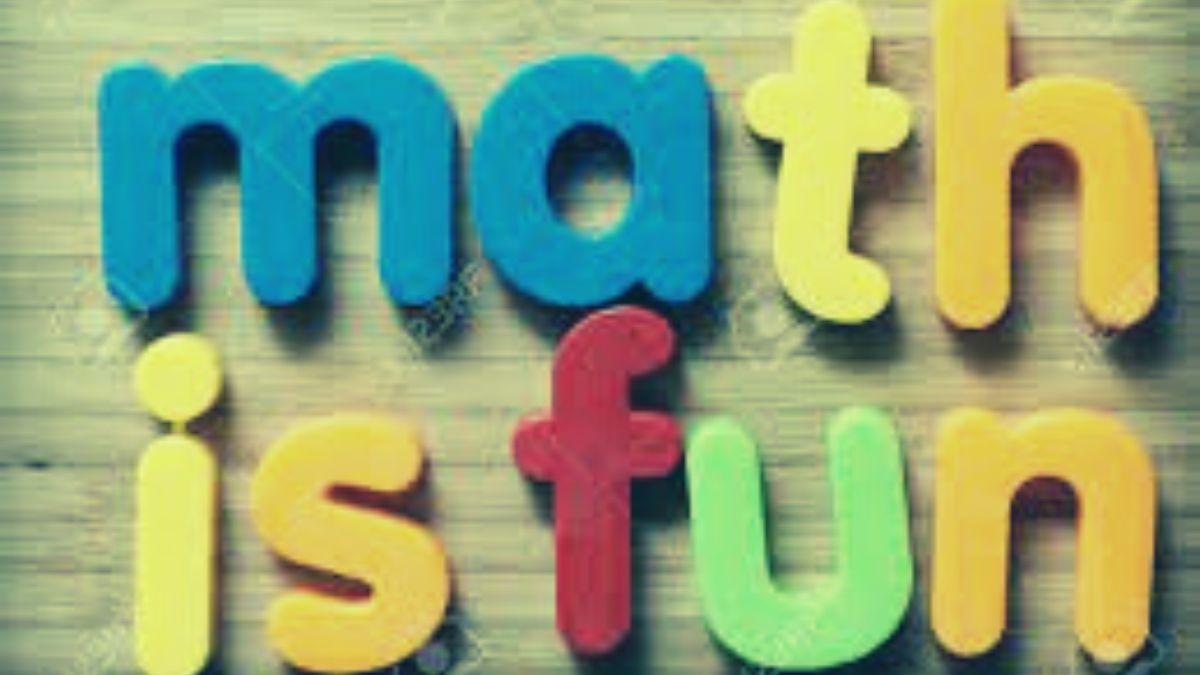# These math riddles are fun, easy, exciting, and what-not!

Do you remember the days when you cursed the subject of “math”? Here we are going to change your harsh perception toward the subject through these exciting math riddles.Math riddles are a great way to get comfortable with the subject. Whether you are a student struggling to like the subject or an adult who would never want to pick your math books again, here we are trying hard to make you like the subject.

Presenting some exciting math riddles.

MATH RIDDLE 1:

Once there lived a honeybee. Unlike many, she was very fond of math. She worked hard and hard, but could not score an A grade in the subject. What do you think would be her grade on the test?

MATH RIDDLE 2:

If 4+2=26, 8+1=79 and 6+5=111. Then, what is 7+3?

MATH RIDDLE 3:

I am a three-digit number. My tens digit is 1 more than my ones digit. My hundreds digit is 1 less than my tens digit. My ones digit is 1 more than 1. Can you guess the number?

MATH RIDDLE 1:

Once there lived a honeybee. Unlike many, she was very fond of math. She worked hard and hard, but could not score an A grade in the subject. What do you think would be her grade on the test?

Answer: The honeybee secured a BEE Plus every time she aimed for an A grade!

MATH RIDDLE 2

If 4+2=26, 8+1=79 and 6+5=111. Then, what is 7+3?

Answer to riddle 7: 410. 4+2=26 is because 4-2=2 and 4+2=6, so it is 26. Therefore, 7-3=4 and 7+3=10(410)

MATH RIDDLE 3:

I am a three-digit number. My tens digit is 1 more than my ones digit. My hundreds digit is 1 less than my tens digit. My ones digit is 1 more than 1. Can you guess the number?